Скачать презентацию MONETARY POLICY Lecture 7 MONETARY THEORY DEMAND FOR

5264d4c244d31b94892306a8c0e7ffe0.ppt

• Количество слайдов: 28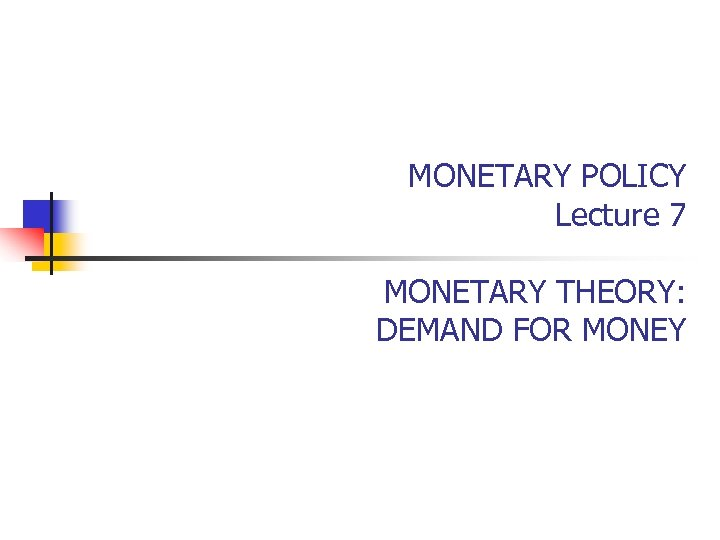MONETARY POLICY Lecture 7 MONETARY THEORY: DEMAND FOR MONEY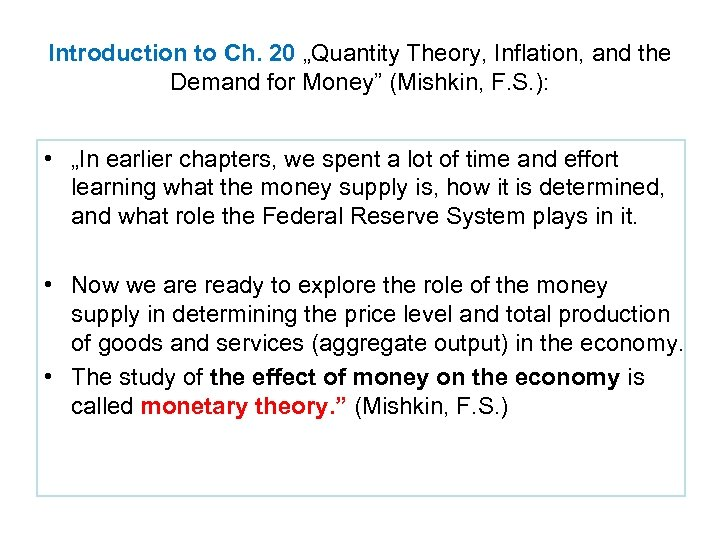Introduction to Ch. 20 „Quantity Theory, Inflation, and the Demand for Money” (Mishkin, F. S. ): • „In earlier chapters, we spent a lot of time and effort learning what the money supply is, how it is determined, and what role the Federal Reserve System plays in it. • Now we are ready to explore the role of the money supply in determining the price level and total production of goods and services (aggregate output) in the economy. • The study of the effect of money on the economy is called monetary theory. ” (Mishkin, F. S. )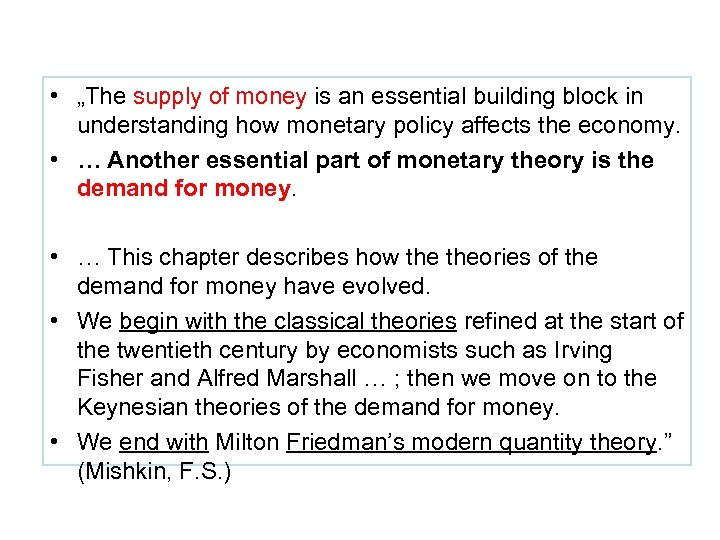• „The supply of money is an essential building block in understanding how monetary policy affects the economy. • … Another essential part of monetary theory is the demand for money. • … This chapter describes how theories of the demand for money have evolved. • We begin with the classical theories refined at the start of the twentieth century by economists such as Irving Fisher and Alfred Marshall … ; then we move on to the Keynesian theories of the demand for money. • We end with Milton Friedman’s modern quantity theory. ” (Mishkin, F. S. )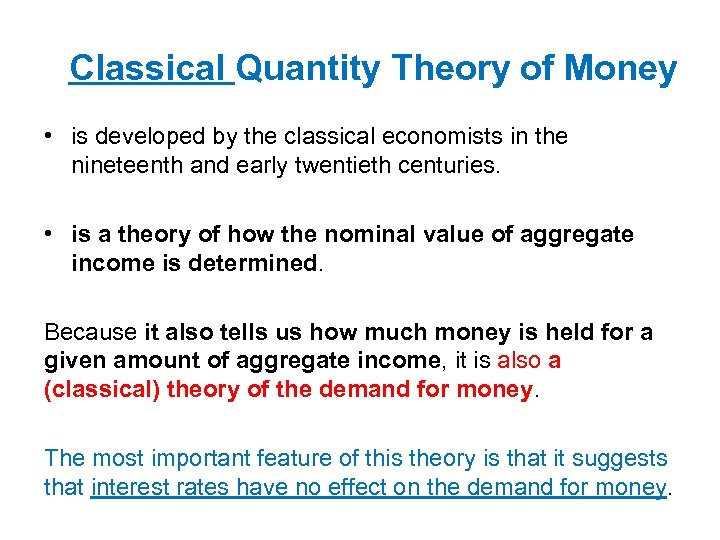Classical Quantity Theory of Money • is developed by the classical economists in the nineteenth and early twentieth centuries. • is a theory of how the nominal value of aggregate income is determined. Because it also tells us how much money is held for a given amount of aggregate income, it is also a (classical) theory of the demand for money. The most important feature of this theory is that it suggests that interest rates have no effect on the demand for money.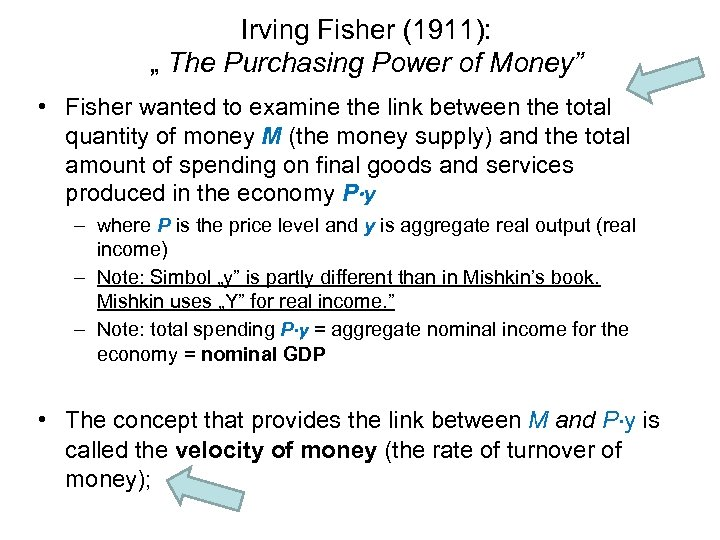Irving Fisher (1911): „ The Purchasing Power of Money” • Fisher wanted to examine the link between the total quantity of money M (the money supply) and the total amount of spending on final goods and services produced in the economy P∙y – where P is the price level and y is aggregate real output (real income) – Note: Simbol „y” is partly different than in Mishkin’s book. Mishkin uses „Y” for real income. ” – Note: total spending P∙y = aggregate nominal income for the economy = nominal GDP • The concept that provides the link between M and P∙y is called the velocity of money (the rate of turnover of money);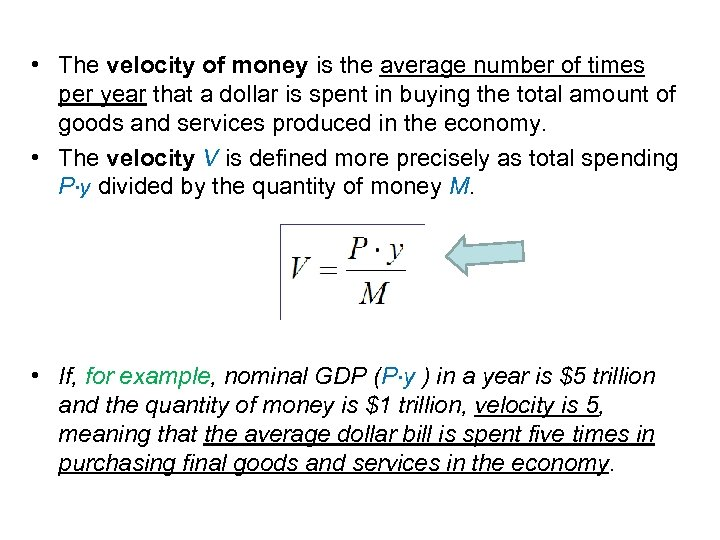• The velocity of money is the average number of times per year that a dollar is spent in buying the total amount of goods and services produced in the economy. • The velocity V is defined more precisely as total spending P∙y divided by the quantity of money M. • If, for example, nominal GDP (P∙y ) in a year is \$5 trillion and the quantity of money is \$1 trillion, velocity is 5, meaning that the average dollar bill is spent five times in purchasing final goods and services in the economy.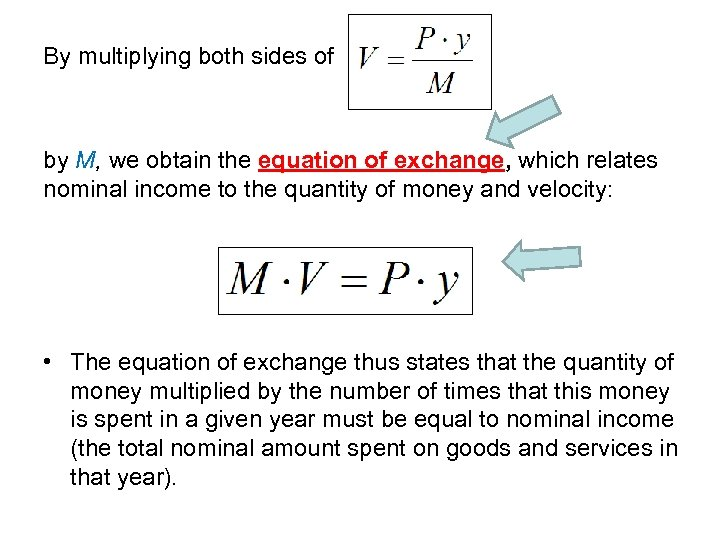By multiplying both sides of by M, we obtain the equation of exchange, which relates nominal income to the quantity of money and velocity: • The equation of exchange thus states that the quantity of money multiplied by the number of times that this money is spent in a given year must be equal to nominal income (the total nominal amount spent on goods and services in that year).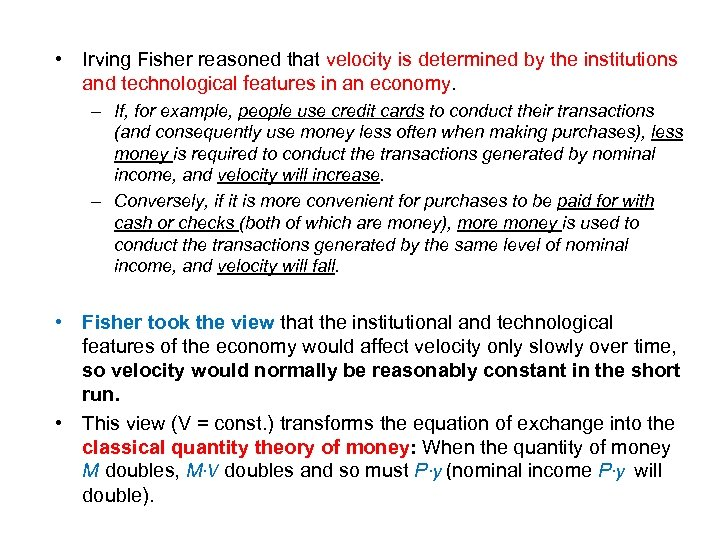• Irving Fisher reasoned that velocity is determined by the institutions and technological features in an economy. – If, for example, people use credit cards to conduct their transactions (and consequently use money less often when making purchases), less money is required to conduct the transactions generated by nominal income, and velocity will increase. – Conversely, if it is more convenient for purchases to be paid for with cash or checks (both of which are money), more money is used to conduct the transactions generated by the same level of nominal income, and velocity will fall. • Fisher took the view that the institutional and technological features of the economy would affect velocity only slowly over time, so velocity would normally be reasonably constant in the short run. • This view (V = const. ) transforms the equation of exchange into the classical quantity theory of money: When the quantity of money M doubles, M∙V doubles and so must P∙y (nominal income P∙y will double).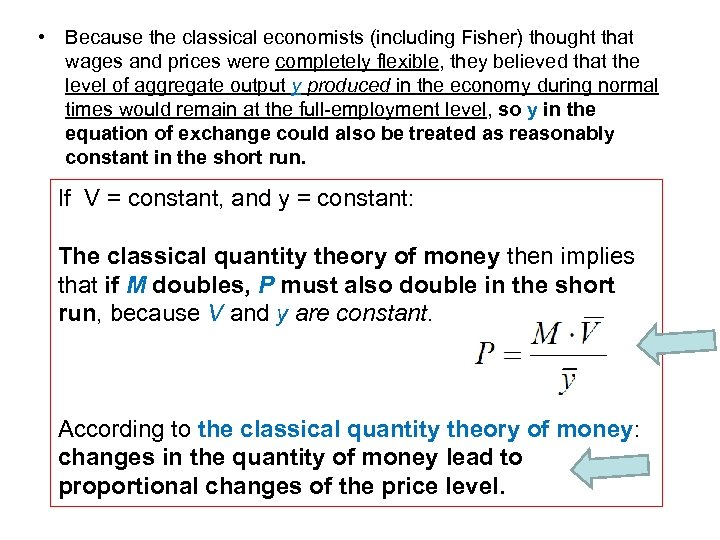• Because the classical economists (including Fisher) thought that wages and prices were completely flexible, they believed that the level of aggregate output y produced in the economy during normal times would remain at the full-employment level, so y in the equation of exchange could also be treated as reasonably constant in the short run. If V = constant, and y = constant: The classical quantity theory of money then implies that if M doubles, P must also double in the short run, because V and y are constant. According to the classical quantity theory of money: changes in the quantity of money lead to proportional changes of the price level.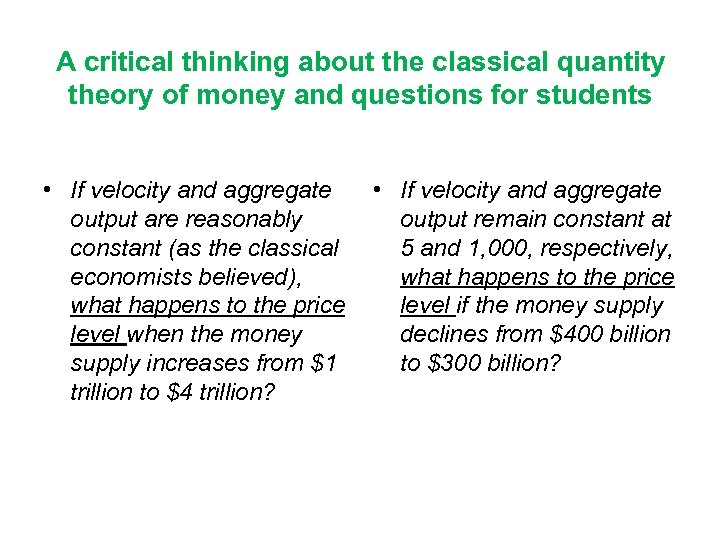A critical thinking about the classical quantity theory of money and questions for students • If velocity and aggregate output are reasonably constant (as the classical economists believed), what happens to the price level when the money supply increases from \$1 trillion to \$4 trillion? • If velocity and aggregate output remain constant at 5 and 1, 000, respectively, what happens to the price level if the money supply declines from \$400 billion to \$300 billion?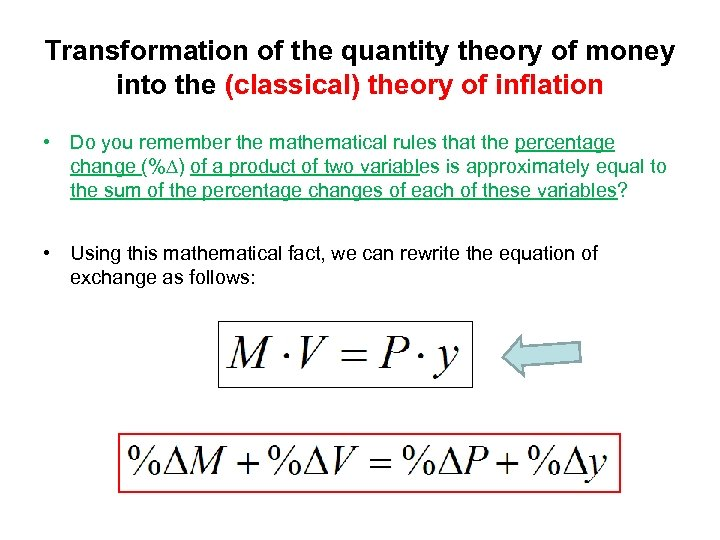Transformation of the quantity theory of money into the (classical) theory of inflation • Do you remember the mathematical rules that the percentage change (%∆) of a product of two variables is approximately equal to the sum of the percentage changes of each of these variables? • Using this mathematical fact, we can rewrite the equation of exchange as follows: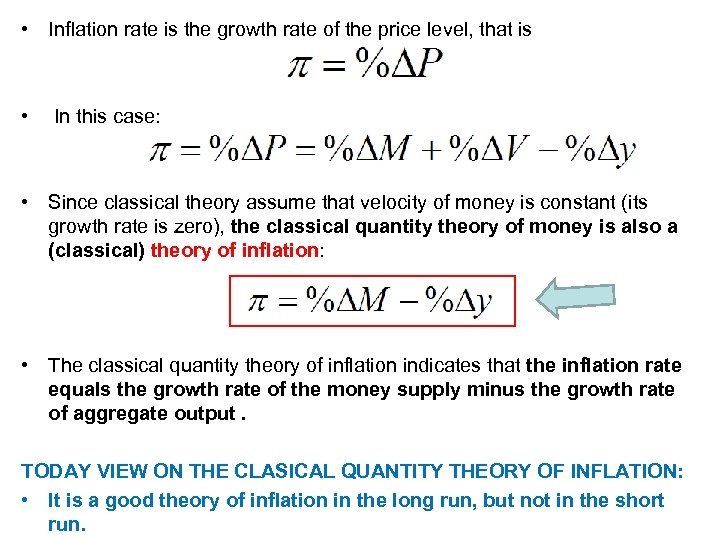• Inflation rate is the growth rate of the price level, that is • In this case: • Since classical theory assume that velocity of money is constant (its growth rate is zero), the classical quantity theory of money is also a (classical) theory of inflation: • The classical quantity theory of inflation indicates that the inflation rate equals the growth rate of the money supply minus the growth rate of aggregate output. TODAY VIEW ON THE CLASICAL QUANTITY THEORY OF INFLATION: • It is a good theory of inflation in the long run, but not in the short run.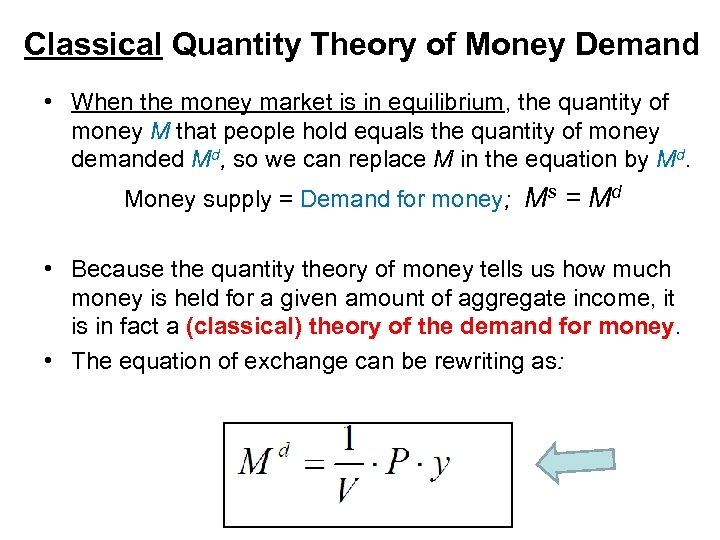Classical Quantity Theory of Money Demand • When the money market is in equilibrium, the quantity of money M that people hold equals the quantity of money demanded Md, so we can replace M in the equation by Md. Money supply = Demand for money; Ms = Md • Because the quantity theory of money tells us how much money is held for a given amount of aggregate income, it is in fact a (classical) theory of the demand for money. • The equation of exchange can be rewriting as: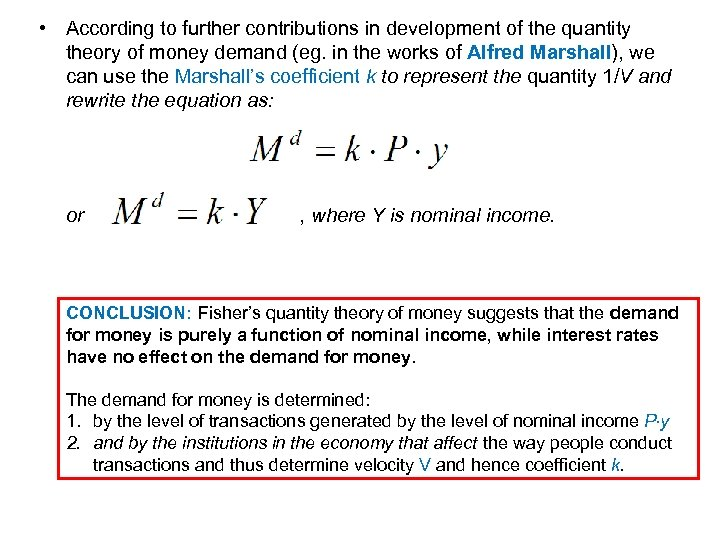• According to further contributions in development of the quantity theory of money demand (eg. in the works of Alfred Marshall), we can use the Marshall’s coefficient k to represent the quantity 1/V and rewrite the equation as: or , where Y is nominal income. CONCLUSION: Fisher’s quantity theory of money suggests that the demand for money is purely a function of nominal income, while interest rates have no effect on the demand for money. The demand for money is determined: 1. by the level of transactions generated by the level of nominal income P∙y 2. and by the institutions in the economy that affect the way people conduct transactions and thus determine velocity V and hence coefficient k.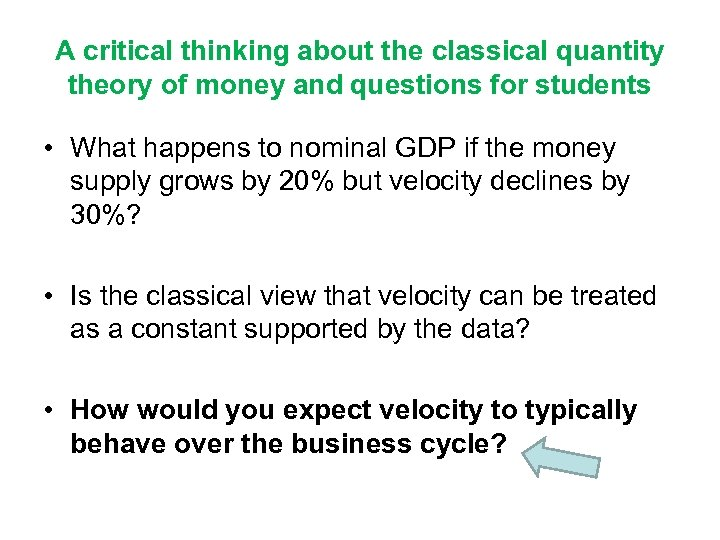A critical thinking about the classical quantity theory of money and questions for students • What happens to nominal GDP if the money supply grows by 20% but velocity declines by 30%? • Is the classical view that velocity can be treated as a constant supported by the data? • How would you expect velocity to typically behave over the business cycle?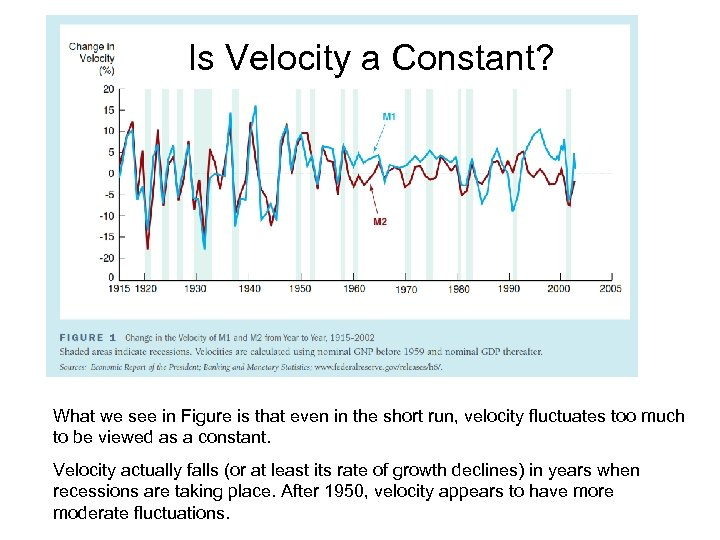Is Velocity a Constant? What we see in Figure is that even in the short run, velocity fluctuates too much to be viewed as a constant. Velocity actually falls (or at least its rate of growth declines) in years when recessions are taking place. After 1950, velocity appears to have more moderate fluctuations.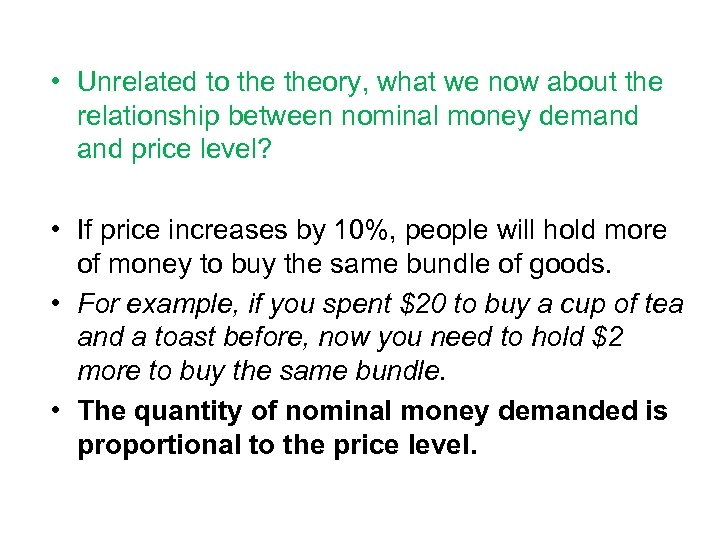• Unrelated to theory, what we now about the relationship between nominal money demand price level? • If price increases by 10%, people will hold more of money to buy the same bundle of goods. • For example, if you spent \$20 to buy a cup of tea and a toast before, now you need to hold \$2 more to buy the same bundle. • The quantity of nominal money demanded is proportional to the price level.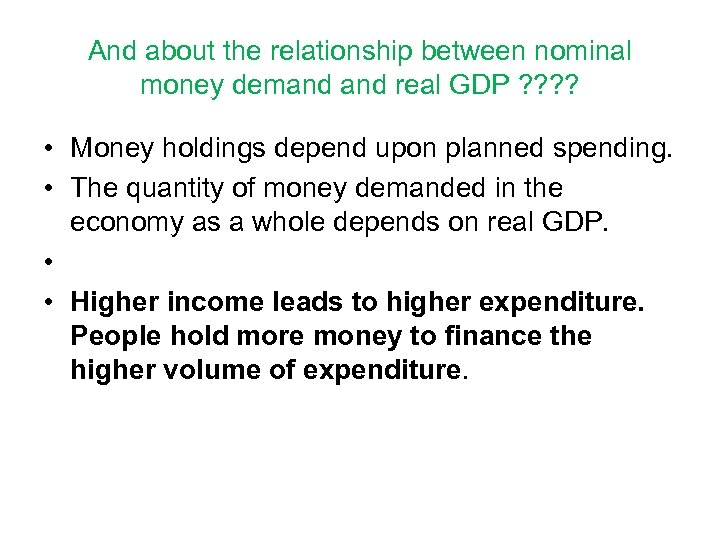And about the relationship between nominal money demand real GDP ? ? • Money holdings depend upon planned spending. • The quantity of money demanded in the economy as a whole depends on real GDP. • • Higher income leads to higher expenditure. People hold more money to finance the higher volume of expenditure.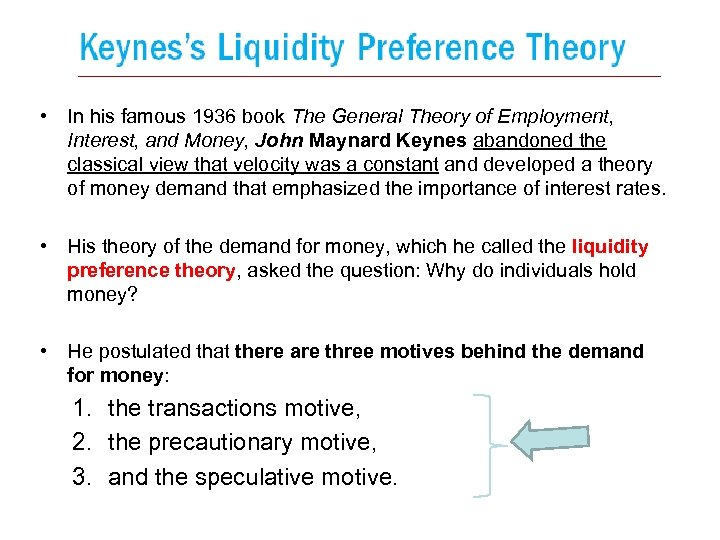• In his famous 1936 book The General Theory of Employment, Interest, and Money, John Maynard Keynes abandoned the classical view that velocity was a constant and developed a theory of money demand that emphasized the importance of interest rates. • His theory of the demand for money, which he called the liquidity preference theory, asked the question: Why do individuals hold money? • He postulated that there are three motives behind the demand for money: 1. the transactions motive, 2. the precautionary motive, 3. and the speculative motive.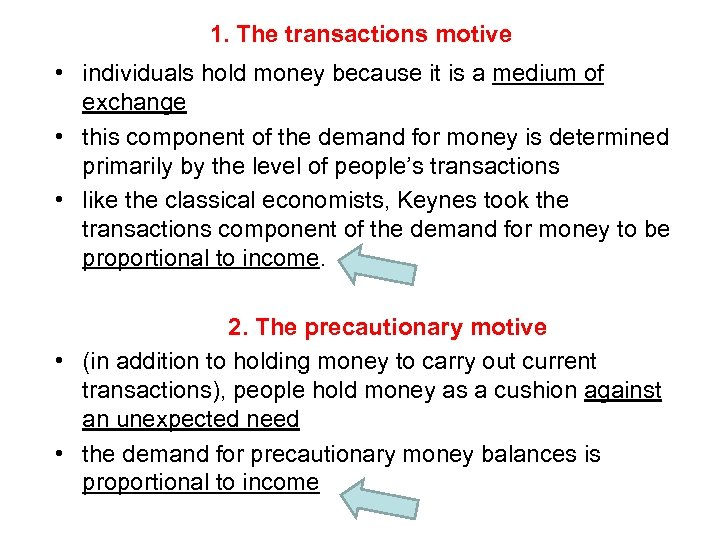1. The transactions motive • individuals hold money because it is a medium of exchange • this component of the demand for money is determined primarily by the level of people’s transactions • like the classical economists, Keynes took the transactions component of the demand for money to be proportional to income. 2. The precautionary motive • (in addition to holding money to carry out current transactions), people hold money as a cushion against an unexpected need • the demand for precautionary money balances is proportional to income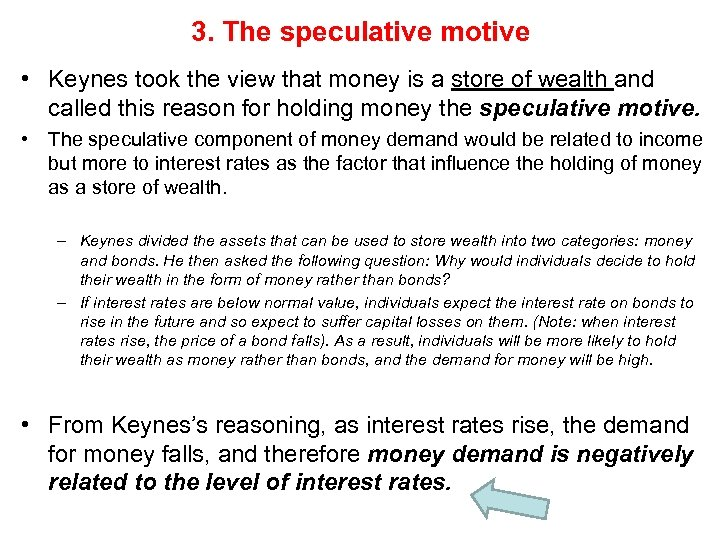3. The speculative motive • Keynes took the view that money is a store of wealth and called this reason for holding money the speculative motive. • The speculative component of money demand would be related to income but more to interest rates as the factor that influence the holding of money as a store of wealth. – Keynes divided the assets that can be used to store wealth into two categories: money and bonds. He then asked the following question: Why would individuals decide to hold their wealth in the form of money rather than bonds? – If interest rates are below normal value, individuals expect the interest rate on bonds to rise in the future and so expect to suffer capital losses on them. (Note: when interest rates rise, the price of a bond falls). As a result, individuals will be more likely to hold their wealth as money rather than bonds, and the demand for money will be high. • From Keynes’s reasoning, as interest rates rise, the demand for money falls, and therefore money demand is negatively related to the level of interest rates.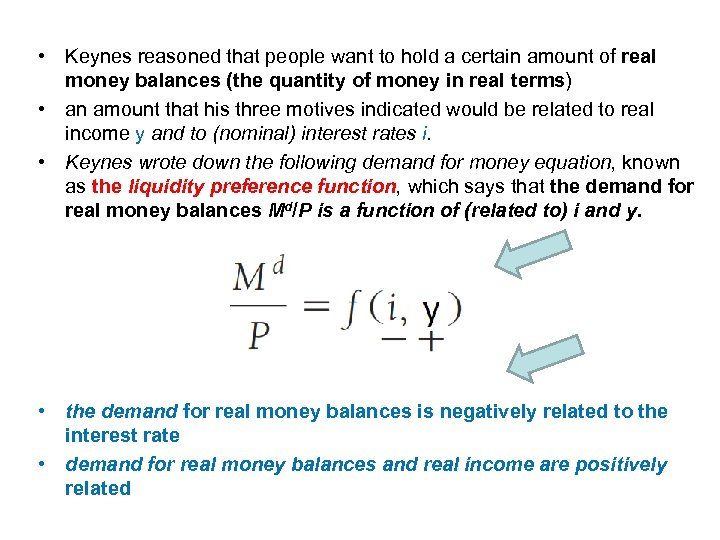• Keynes reasoned that people want to hold a certain amount of real money balances (the quantity of money in real terms) • an amount that his three motives indicated would be related to real income y and to (nominal) interest rates i. • Keynes wrote down the following demand for money equation, known as the liquidity preference function, which says that the demand for real money balances Md/P is a function of (related to) i and y. • the demand for real money balances is negatively related to the interest rate • demand for real money balances and real income are positively related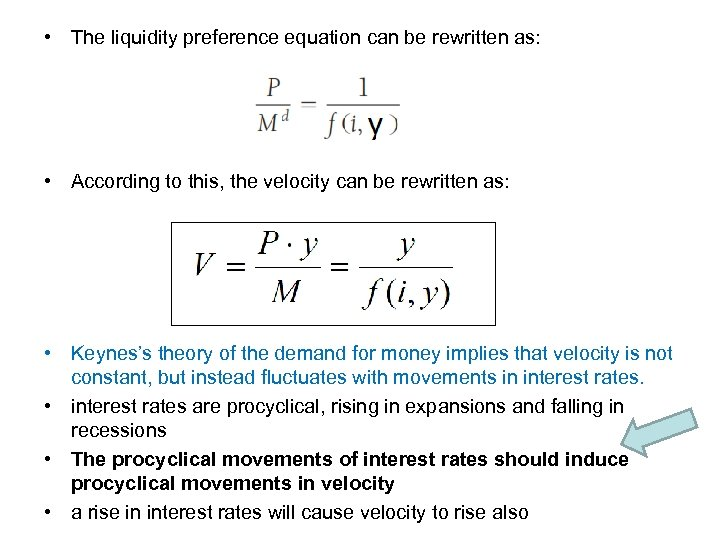• The liquidity preference equation can be rewritten as: • According to this, the velocity can be rewritten as: • Keynes’s theory of the demand for money implies that velocity is not constant, but instead fluctuates with movements in interest rates. • interest rates are procyclical, rising in expansions and falling in recessions • The procyclical movements of interest rates should induce procyclical movements in velocity • a rise in interest rates will cause velocity to rise also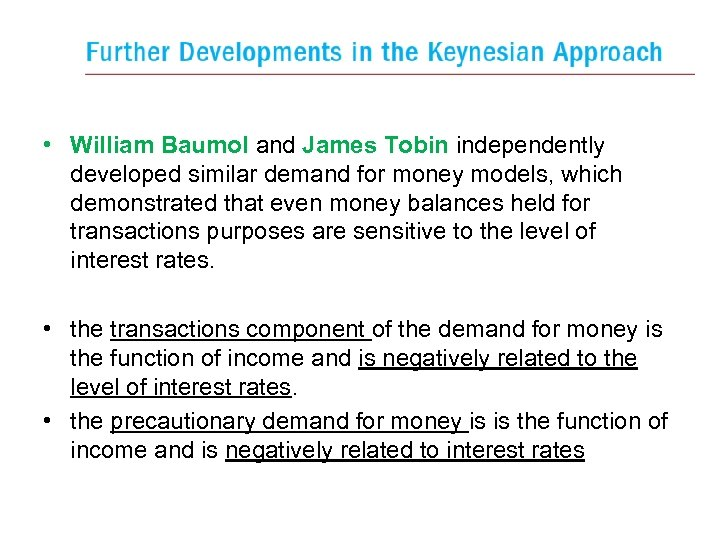• William Baumol and James Tobin independently developed similar demand for money models, which demonstrated that even money balances held for transactions purposes are sensitive to the level of interest rates. • the transactions component of the demand for money is the function of income and is negatively related to the level of interest rates. • the precautionary demand for money is is the function of income and is negatively related to interest rates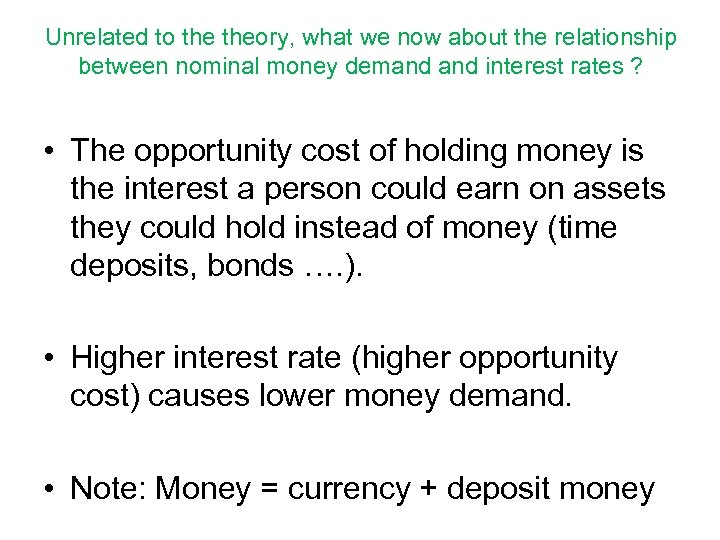Unrelated to theory, what we now about the relationship between nominal money demand interest rates ? • The opportunity cost of holding money is the interest a person could earn on assets they could hold instead of money (time deposits, bonds …. ). • Higher interest rate (higher opportunity cost) causes lower money demand. • Note: Money = currency + deposit money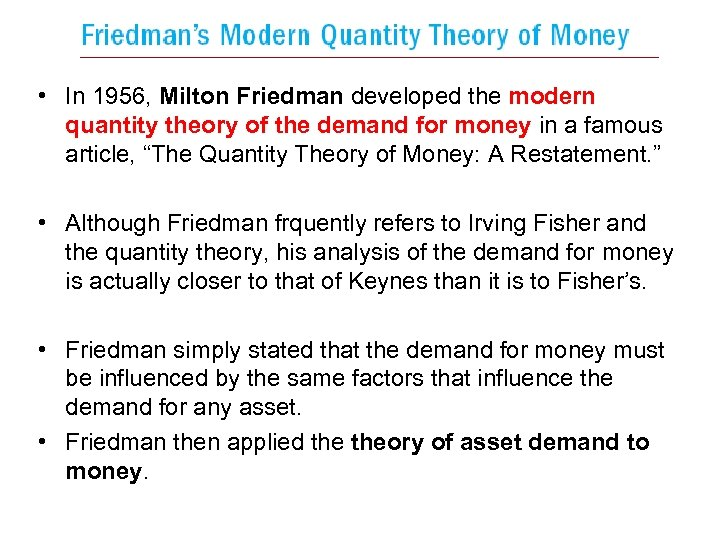• In 1956, Milton Friedman developed the modern quantity theory of the demand for money in a famous article, “The Quantity Theory of Money: A Restatement. ” • Although Friedman frquently refers to Irving Fisher and the quantity theory, his analysis of the demand for money is actually closer to that of Keynes than it is to Fisher’s. • Friedman simply stated that the demand for money must be influenced by the same factors that influence the demand for any asset. • Friedman then applied theory of asset demand to money.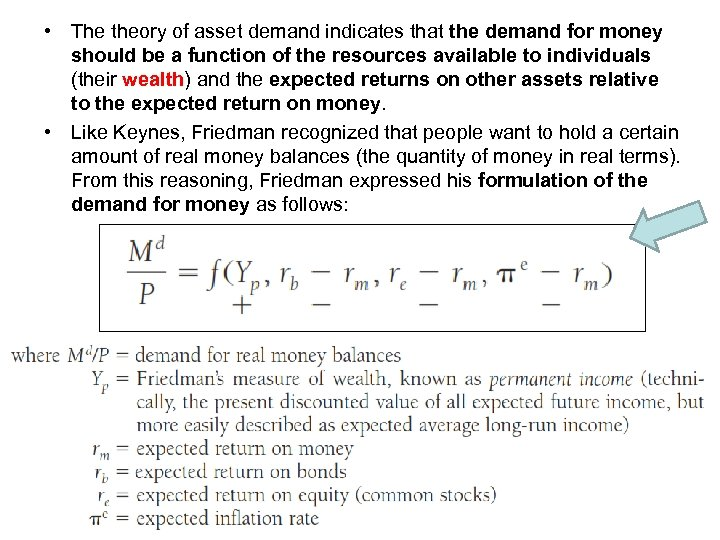• The theory of asset demand indicates that the demand for money should be a function of the resources available to individuals (their wealth) and the expected returns on other assets relative to the expected return on money. • Like Keynes, Friedman recognized that people want to hold a certain amount of real money balances (the quantity of money in real terms). From this reasoning, Friedman expressed his formulation of the demand for money as follows: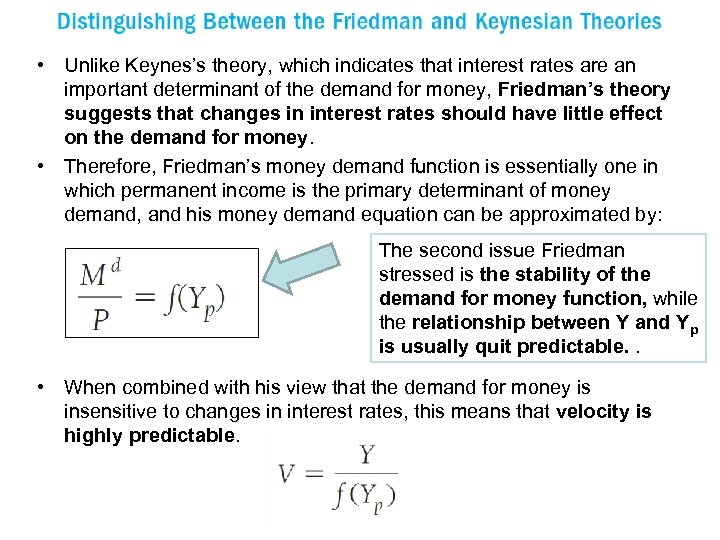• Unlike Keynes’s theory, which indicates that interest rates are an important determinant of the demand for money, Friedman’s theory suggests that changes in interest rates should have little effect on the demand for money. • Therefore, Friedman’s money demand function is essentially one in which permanent income is the primary determinant of money demand, and his money demand equation can be approximated by: The second issue Friedman stressed is the stability of the demand for money function, while the relationship between Y and Yp is usually quit predictable. . • When combined with his view that the demand for money is insensitive to changes in interest rates, this means that velocity is highly predictable.